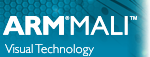OpenGL ES SDK for Android ARM Developer Center
Matrix.cpp File Reference
`#include "Matrix.h"`

Go to the source code of this file.

## Functions

void matrixIdentityFunction (float *matrix)
Takes a 4 * 4 and sets the elements to the Identity function. More...

void matrixTranslate (float *matrix, float x, float y, float z)
Takes in a 4 * 4 matrix and translates it by the vector defined by x y and z. More...

void matrixMultiply (float *destination, float *operand1, float *operand2)
Takes 2 matrices and multiplies them together. Then stores the result in a third matrix. More...

void matrixFrustum (float *matrix, float left, float right, float bottom, float top, float zNear, float zFar)
Create a viewing frustum and store the result in the first parameter. This is usually called by matrixPerspective. More...

void matrixPerspective (float *matrix, float fieldOfView, float aspectRatio, float zNear, float zFar)
Create a perspective projection matrix and store the results in the first parameter. More...

void matrixRotateX (float *matrix, float angle)
Rotates a matrix around the x axis by a given angle. More...

void matrixRotateY (float *matrix, float angle)
Rotates a matrix around the y axis by a given angle. More...

void matrixRotateZ (float *matrix, float angle)
Rotates a matrix around the Z axis by a given angle. More...

void matrixScale (float *matrix, float x, float y, float z)
Scales a matrix by a given factor in the x, y and z axis. More...

Function to convert degrees into Radians. More...

## Function Documentation

 float matrixDegreesToRadians ( float degrees )

Function to convert degrees into Radians.

Parameters
 [in] degrees Angle to be converted.
Returns

Definition at line 152 of file Matrix.cpp.

 void matrixFrustum ( float * matrix, float left, float right, float bottom, float top, float zNear, float zFar )

Create a viewing frustum and store the result in the first parameter. This is usually called by matrixPerspective.

Parameters
 [out] matrix Destination of the frustum. [in] left Left side of the frustum. [in] right Right side of the frustum. [in] bottom Bottom of the frustum. [in] top Top of the frustum. [in] zNear Near visible distance of the frustum. [in] zFar Far visible distance of the frustum.

Definition at line 78 of file Matrix.cpp.

 void matrixIdentityFunction ( float * matrix )

Takes a 4 * 4 and sets the elements to the Identity function.

Parameters
 [out] matrix Pointer to an array of a 4 * 4 matrix. Must be at least 16 elements long.

Definition at line 23 of file Matrix.cpp.

 void matrixMultiply ( float * destination, float * operand1, float * operand2 )

Takes 2 matrices and multiplies them together. Then stores the result in a third matrix.

Parameters
 [out] destination A pointer to the array the result of the multiplication should be stored. [in] operand1 A pointer to the first matrix to be multiplied. [in] operand2 A pointer to the second matrix to be multiplied.

Definition at line 58 of file Matrix.cpp.

 void matrixPerspective ( float * matrix, float fieldOfView, float aspectRatio, float zNear, float zFar )

Create a perspective projection matrix and store the results in the first parameter.

Parameters
 [out] matrix Destination of the perspective projection matrix. [in] fieldOfView The field of view in degrees. How far you expect the camera to see in degrees. [in] aspectRatio The aspect ratio of your viewport. [in] zNear How close objects can be to the camera before they disappear. [in] zFar How far away objects can be before they are no longer drawn.

Definition at line 96 of file Matrix.cpp.

 void matrixRotateX ( float * matrix, float angle )

Rotates a matrix around the x axis by a given angle.

Parameters
 [in,out] matrix A pointer to the matrix to be rotated [in] angle A float representing the angle to rotate by in degrees.

Definition at line 104 of file Matrix.cpp.

 void matrixRotateY ( float * matrix, float angle )

Rotates a matrix around the y axis by a given angle.

Parameters
 [in,out] matrix A pointer to the matrix to be rotated [in] angle A float representing the angle to rotate by in degrees.

Definition at line 116 of file Matrix.cpp.

 void matrixRotateZ ( float * matrix, float angle )

Rotates a matrix around the Z axis by a given angle.

Parameters
 [in,out] matrix A pointer to the matrix to be rotated [in] angle A float representing the angle to rotate by in degrees.

Definition at line 128 of file Matrix.cpp.

 void matrixScale ( float * matrix, float x, float y, float z )

Scales a matrix by a given factor in the x, y and z axis.

Parameters
 [in,out] matrix A pointer to the matrix to be scaled. [in] x Scaling factor in the X axis. [in] y Scaling factor in the Y axis. [in] z Scaling factor in the Z axis.

Definition at line 140 of file Matrix.cpp.

 void matrixTranslate ( float * matrix, float x, float y, float z )

Takes in a 4 * 4 matrix and translates it by the vector defined by x y and z.

Parameters
 [in,out] matrix Pointer to an array of a 4 * 4 matrix. Must be at least 16 elements long [in] x X component of the translation vector. [in] y Y component of the translation vector. [in] z Z component of the translation vector.

Definition at line 48 of file Matrix.cpp.